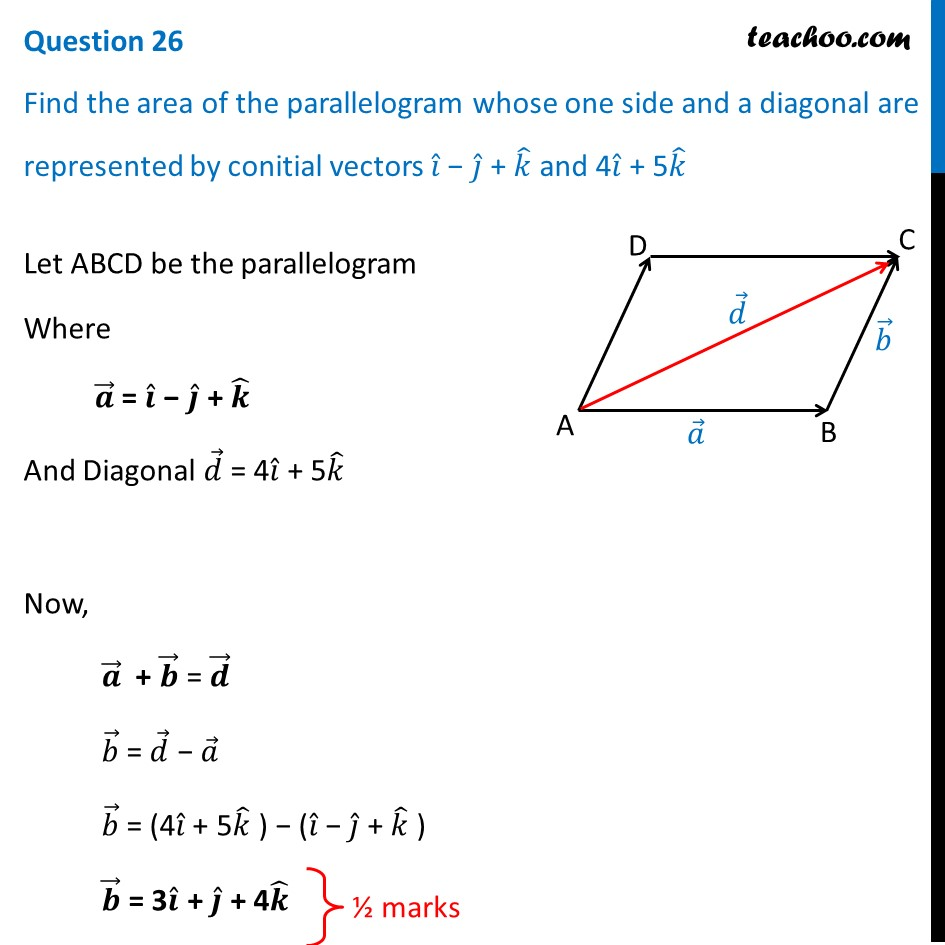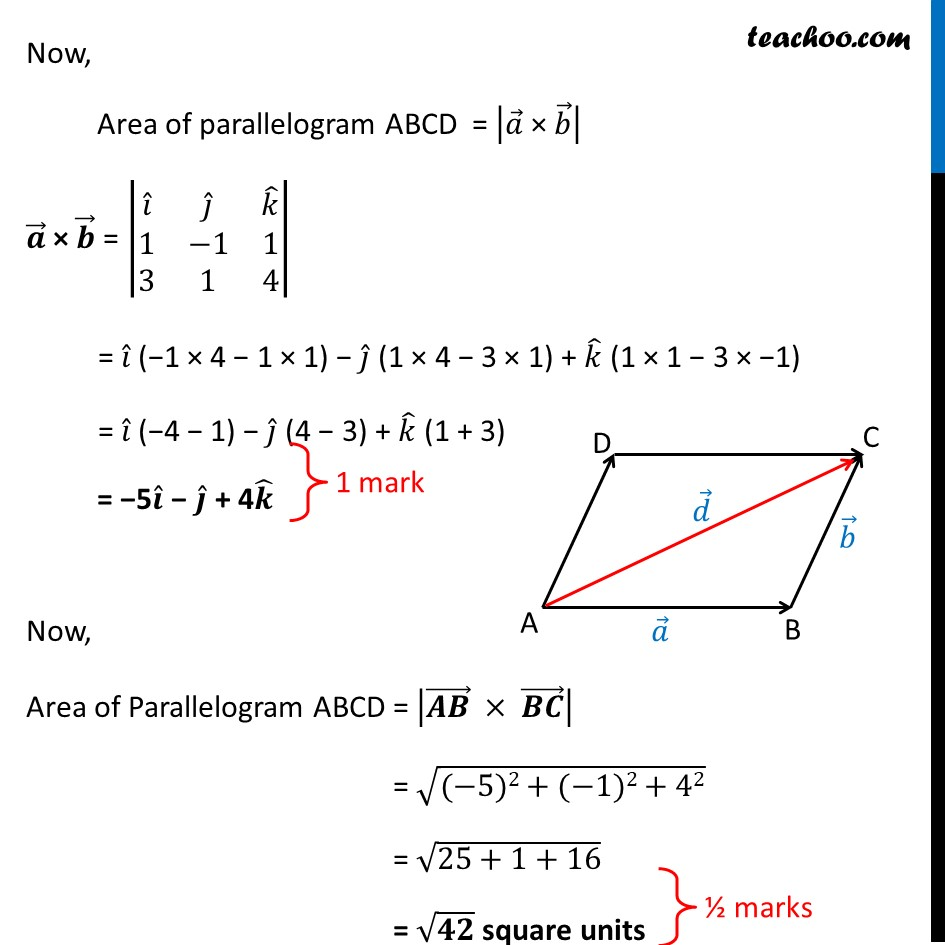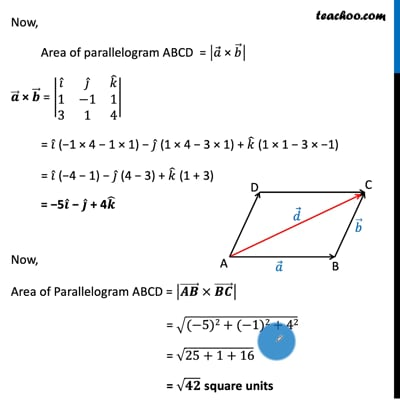CBSE Class 12 Sample Paper for 2021 Boards

Class 12
Solutions of Sample Papers and Past Year Papers - for Class 12 Boards

## Find the area of the parallelogram whose one side and a diagonal are represented by conitial vectors i ̂ - j ̂ + k ̂ and 4i ̂ + 5k ̂This video is only available for Teachoo black users

Introducing your new favourite teacher - Teachoo Black, at only ₹83 per month

### Transcript

Question 26 Find the area of the parallelogram whose one side and a diagonal are represented by conitial vectors 𝑖 ̂ − 𝑗 ̂ + 𝑘 ̂ and 4𝑖 ̂ + 5𝑘 ̂ Let ABCD be the parallelogram Where 𝒂 ⃗ = 𝒊 ̂ − 𝒋 ̂ + 𝒌 ̂ And Diagonal 𝑑 ⃗ = 4𝑖 ̂ + 5𝑘 ̂ Now, 𝒂 ⃗ + 𝒃 ⃗ = 𝒅 ⃗ 𝑏 ⃗ = 𝑑 ⃗ − 𝑎 ⃗ 𝑏 ⃗ = (4𝑖 ̂ + 5𝑘 ̂ ) − (𝑖 ̂ − 𝑗 ̂ + 𝑘 ̂ ) 𝒃 ⃗ = 3𝒊 ̂ + 𝒋 ̂ + 4𝒌 ̂ Now, Area of parallelogram ABCD = |𝑎 ⃗" × " 𝑏 ⃗ | 𝒂 ⃗ × 𝒃 ⃗ = |■8(𝑖 ̂&𝑗 ̂&𝑘 ̂@1&−1&1@3&1&4)| = 𝑖 ̂ (−1 × 4 − 1 × 1) − 𝑗 ̂ (1 × 4 − 3 × 1) + 𝑘 ̂ (1 × 1 − 3 × −1) = 𝑖 ̂ (−4 − 1) − 𝑗 ̂ (4 − 3) + 𝑘 ̂ (1 + 3) = −5𝒊 ̂ − 𝒋 ̂ + 4𝒌 ̂ Now, Area of Parallelogram ABCD = |(𝑨𝑩) ⃗ × (𝑩𝑪) ⃗ | = √((−5)2+(−1)2+42) = √(25+1+16) = √𝟒𝟐 square units